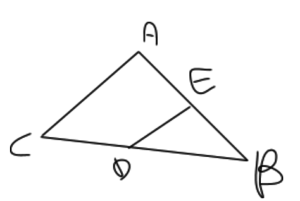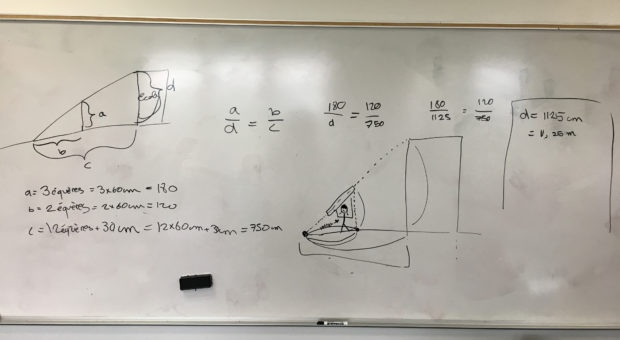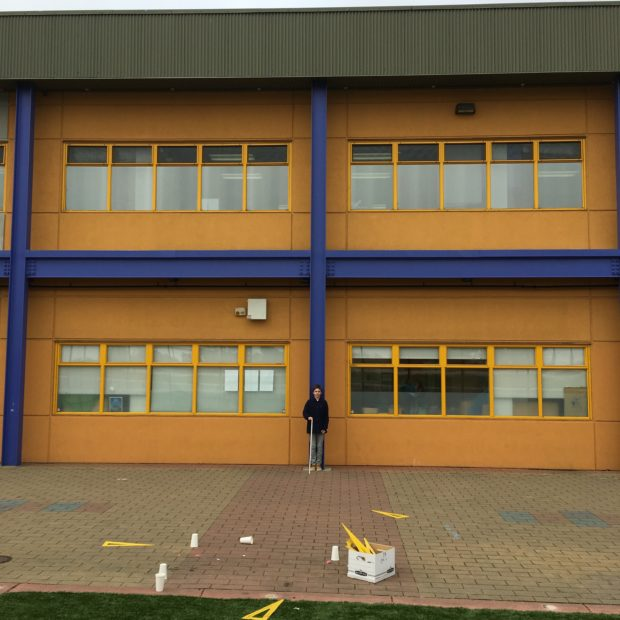# Guest Blogger: The Thales Theorem, by 8th Grader Klavie G.Klavie G. explains how she applied the Thales theorem that she learned in 8th grade math class to solve a problem in the real world.

In math class we learned how to use the Thales theorem. The Thales theorem is a theorem that helps us determine the length of a line in a triangle.

For the figure below, the property would be: BE/BA= BD/BC= ED/COur class decided to measure the height of the school using the Thales theorem.After having done our calculations (image above) we determined that the building measured 11.25 metres.

To be sure of our calculations we applied a different method by using proportionality. We placed a student in front of the building and then we photoshopped the picture we took to see how many times we could see the student in front of the school.We can see the student 5 and ½ times. His height is about 1m 62. So the height of the building is approximately 10 meters.

With the calculations we found the height of the school to be 11.25 meters and by using proportionality we found 10 meters. We were pleased when we found that both our measurements were fairly close and it gave us a good idea of the building's height.

*In 2020, the International School of the Peninsula (ISTP) formally changed its name to Silicon Valley International School (INTL) to better reflect its bilingual programs, location, and international values.